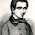## Tuesday, July 3, 2018

### The world is geometrical

In my anti-positivist counterfactual history, here is the first axiom.

Geometry axiom: The world is geometrical.

The most familiar geometry is Euclidean geometry, where the world is R3 and distance squared is given by dx2 + dy2 + dz2. There are other geometries.

Newtonian mechanics is a geometrical theory. Space is represented by R3, with Euclidean metric. That is a geometrical object because of the linear structure and the metric. Lines can be defined as the short distance between points. Plane, circles, triangles, and other geometric objects can be defined.

It is also a geometrical object because there is a large class of transformations that preserve the metric, and hence also the lines and circles determined by the metric. Those transformations are the rotations, reflections, and translations. For example, the transformation (x,y,z) → (x+5,y,z) preserves distances, and takes lines to lines and circles to circles.

Mathematically, a geometry can be defined in terms of some structure like a metric, or the transformations preserving that structure. This view has been known as the Klein Erlangen program since 1872.

The laws of classical equations can be written in geometrical equations like F=ma, where F is the force vector, a is the acceleration vector, and m is the mass. All are functions on Euclidean space. What makes F=ma geometrical is not just that it is defined on a geometrical space, or that vectors are used. The formula is geometrical because all quantities are covariant under the relevant transformations.

Classical mechanics does not specify where you put your coordinate origin (0,0,0), or how the axes are oriented. You can make any choice, and then apply one of the symmetries of Euclidean space. Formulas like F=ma will look the same, and so will physical computations. You can even do a change of coordinates that does not preserve the Euclidean structure, and covariance will automatically dictate the expression of the formula in those new coordinates.

One can think of Euclidean space and F=ma abstractly, where the space has no preferred coordinates, and F=ma is defined on that abstract space. Saying that F=ma is covariant with respect to a change of coordinates is exactly the same as saying F=ma is well-defined on a coordinate-free Euclidean space.

The strongly geometrical character of classical mechanics was confirmed by a theorem of Neother that symmetries are essentially the same as conservation laws. Momentum is conserved because spacetime has a spatial translational symmetry, energy is conserved because of time translation symmetry, and angular momentum is conserved because of rotational symmetry.

Next, we look at geometries that make physical sense.

1.I would change your axiom "Geometry axiom: The world is geometrical." to "Geometry axiom: The world looks geometrical."

2.It's not so much geometry but coordinate-free geometry that moved things ahead, although most college students could not even tell you the difference between a spinor and a tensor these days, let alone what is Clifford algebra. Poincaré was proven right that geometric systems are mere convention and that non-Euclidean geometry can be translated back into Euclidean geometry. Granted, it may not be simple to understand something like the Nash embedding theorem but a model is about convenience. Projective geometry came from adding idealized points to Euclidean geometry and has Euclidean analogs, though one can derive the geometries from projective geometry. As an example of convenience, special relativity can be well represented using hyperbolic geometry: "The Special Theory of Relativity, which received its initial formulation by Poincaré and Einstein in 1905, gained general acceptance in 1908 about the same time as Minkowski's interpretation in terms of the 4 dimensional world Soon after, in the years 1910-1914, the Yugoslav mathematician Vladimir Varićak showed that this theory finds a natural interpretation in hyperbolic (or Bolyai-Lobachevski) geometry, an idea also put forward in less detail by a few other writers about the same time, notably Robb (1910, etc.) and Borel (1913). Despite its apparently fundamental nature, this hyperbolic interpretation remains little known and has not yet found its way into standard texts on relativity theory, even after nearly a century." https://arxiv.org/pdf/1102.0462.pdf
https://londmathsoc.onlinelibrary.wiley.com/doi/pdf/10.1112/blms/3.3.257

3.It should be noted that Newton's Principia was made with geometric arguments, inspired by Euclid.

It's amazing how undergraduate curriculums have included so little geomtery and even the MAA has noted how much it has fallen out of high schools: "Every mathematics major program should include substantial geometric content. As pointed out in many documents over the past twenty-five years, instruction in geometric mathematics has suffered from neglect in the recent past. With the 'push to calculus,' many students never recover from a high school education that skips too quickly over geometry. This must be remedied. Geometry, in a centuries-long interplay with algebra, is one of the vital halves of mathematics, and this is just as true today as in ancient times."
https://www.maa.org/sites/default/files/Geometry.pdf

High school gets you to about 1500 and college about 1700. Mathematics is another postmodern humanities subject where they obsess over arbitrary axioms of set theory or baby stuff. They think teaching a few axioms or definitions implies they taught you large areas of derived and applied mathematics but it's a revisionist account of history where they get everything backwards. Generalizations came later and specifics came first. What idiotic idealism. They just belabor every little result with a thousand excercises full of busy work and then complain they "don't have enough time."

For instance, Terry Tao, a world-class mathematician, even teaches a dumbed-down linear algebra course. You couldn't survive a fifth-rate engineering, computer science or physics course with this kind of measly coverage. Ridiculous! Here is a comment on a sample final: "Note: 'Kernel' is the same thing as 'Null space'. For the purposes of this class, the field of scalars F can always taken to be the real numbers R." http://www.math.ucla.edu/~tao/resource/general/115a.3.02f/

I see linear algebra courses in India cover twice as much as Tao.